Courses

# Notes | EduRev

## NEET : Notes | EduRev

The document Notes | EduRev is a part of the NEET Course Physics 28 Years Past year papers for NEET/AIPMT Class 12.
All you need of NEET at this link: NEET

Q.1. Which of the following acts as a circuit protection device?    (2019)
A: Conductor
B: Inductor
C: Switch
D: Fuse
Ans:
D
Solution:

Fuse wire has less melting point so when excess current  flows, due to heat produced in it, it melts.

Q.2. A parallel plate capacitor of capacitance 20 mF is being charged by a voltage source whose potential is changing at the rate of 3 V/s. The conduction current through the connecting wires, and the displacement current through the plates of the capacitor, would be, respectively.    (2019)
A: Zero, 60 μA
B: 60 μA, 60 μA
C: 60 μA, zero
D: Zero, zero
Ans:
B
Solution:

Capacitance of capacitor C = 20 mF
= 20 × 10-6 F
Rate of change of potential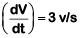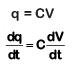ic = 20 × 10–6 × 3
= 60 × 10–6 A
= 60 μA
As we know that id = ic = 60 mA

Q.3. The magnetic potential energy stored in a certain inductor is 25 mJ, when the current in the inductor is 60 mA. This inductor is of inductance :-    (2018)
A: 0.138 H
B: 138.88 H
C: 1.389 H
D: 13.89 H
Ans:
D
Solution:

Energy stored in the inductor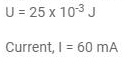Energy stored in the inductor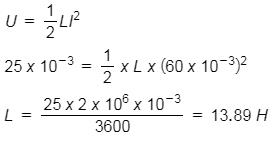Q.4. In the circuit shown in the figure, the input voltage Vi is 20 V, VBE = 0 and VCE = 0. The values of IB, IC and β are given by :-    (2018)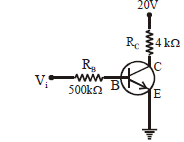A: IB = 40 µA, IC = 10 mA, β = 250
B: IB = 25 µA, IC = 5 mA, β = 200
C: IB = 20 µA, IC = 5 mA, β = 250
D: IB = 40 µA, IC = 5 mA, β = 125
Ans:
D
Solution: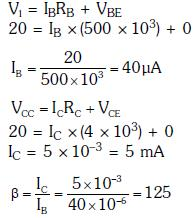Q.5. An inductor 20 mH, a capacitor 100 μF and a resistor 50 Ω are connected in series across a source of emf, V = 10 sin 314 t. The power loss in the circuit is     (2018)
A: 0.79 W
B: 0.43 W
C: 2.74 W
D: 1.13 W
Ans:
A
Solution: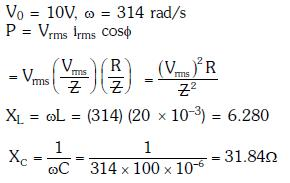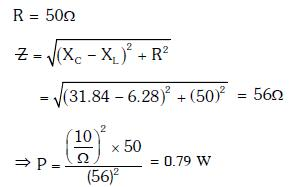Q.6. Figure shows a circuit that contains three identical resistors with resistance R = 9.0 Ω each, two identical inductors with inductance L = 2.0 m Heach, and an ideal battery with emf ε = 18 V. The current 'i' through the battery just after the switch closed is,...... :-      (2017)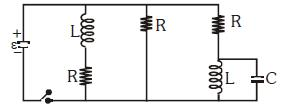A: 0.2 A
B: 2 A
C: 0 ampere
D: 2 mA
Ans:
B
Solution: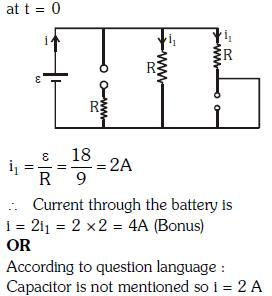Q.7. An inductor 20 mH, a capacitor 50 μF and a resistor. 40Ω are connected in series across a source of emf V = 10 sin 340 t. The power loss in A.C. circuit is     (2016)
A: 0.89W
B: 0.51W
C: 0.67W
D: 0.76W
Ans:
B
Solution: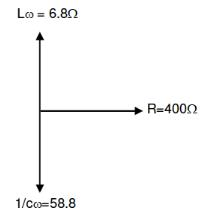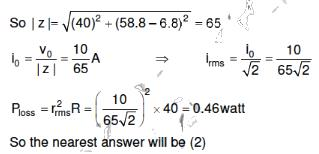Q.8. A small signal voltage V(t) = V0 sinωt is applied across an ideal capacitor C :    (2016)
A: Current I(t), leads voltage V(t) by 180 degree
B: Current I(t), lags voltage V(t) by 90 degree
C: Over a full cycle the capacitor C does not consume any energy from the voltage source.
D: Current I(t) is in phase with voltage V(t)
Ans:
C
Solution:

Capacitor does not consume energy effectively over full cycles

Q.9. A resistance 'R' draws power 'P' when connected to an AC source. If an inductance is now placed in series with the resistance, such that the impedance of the circuit becomes 'Z', the power drawn will be :
A: P
B: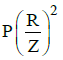C: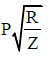D: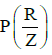Ans: B
Solution:

A resistance R draws power P when connected to an AC source.
The magnitude of voltage of the AC source is
V2 = RP
∴ V=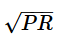An inductor of inductance L and reactance ωL is now placed in series with the resistance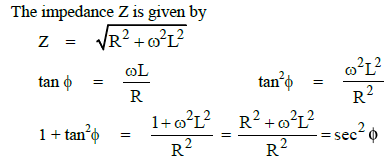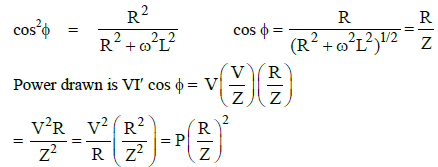Q.10. A transformer having efficiency of 90% is working on 200 V and 3 kW power supply. If the current in the secondary coil is 6A, the voltage across the secondary coil and the current in the primary coil respectively are :    (2014)
A: 450 V, 13.5 A
B: 600 V, 15 A
C: 300 V, 15 A
D: 450 V, 15 A

Ans: D
Solution:
Initial power = 3000 W
As the efficiency is 90%, then final power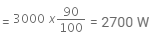That is,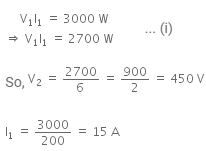Offer running on EduRev: Apply code STAYHOME200 to get INR 200 off on our premium plan EduRev Infinity!

14 docs|25 tests

,

,

,

,

,

,

,

,

,

,

,

,

,

,

,

,

,

,

,

,

,

;